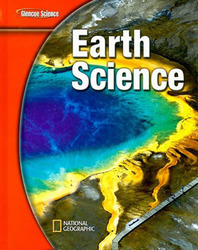# Glencoe Earth Science

### Math Practice

Unit 1: Earth Materials
Chapter 2 - Calculating Density
Chapter 4 - Calculate Thickness

Unit 2: The Changing Surface of Earth
Chapter 7 - Calculate Percentages
Chapter 9 - Calculate Rate of Flow

Unit 3: Earth's Internal Processes
Chapter 11 - Find a Ratio
Chapter 12 - Calculate Percent

Unit 4: Change and Earth's History
Chapter 14 - Calculate Percentages

Unit 5: Earth's Air and Water
Chapter 18 - Calculate Density
Chapter 19 - Find the Slope

Unit 6: You and the Environment
Chapter 21 - Calculate Percentages

Unit 7: Astronomy
Chapter 22 - Make and Use Graphs
Chapter 24 - Use PercentagesScience HomeProduct InfoSite MapContact UsPlease read our Terms of Use and Privacy Policy before you explore our Web site. To report a technical problem with this Web site, please contact the Web Producer.